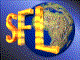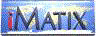| iMatix home page | << | < | > | >>SFLVersion 2.11

### http_decode_meta

```#include "sflhttp.h"
size_t
http_decode_meta (
char  *output,
char **input,
size_t outmax)
```

#### Synopsis

Translates special characters from HTML/SGML metacharacters. The input buffer is not modified; you supply an output buffer and specify the maximum size of this buffer. The input buffer must end in a null. The two buffers may be the same, since decoding reduces the size of the data. Returns the final size of the translated data excluding the final null byte. If the resulting data is too long, it is brutally truncated. Invalid meta-characters are left as-is. Normally the input pointer points to the final null; however if the input string runs out in the middle of a meta-character, the input pointer is left pointing at the start of that meta-character (the '&'). If the two buffers are the same, it may be the case that this character has been overwritten with the terminating null.

#### Source Code - (sflhttp.c)

```{
char
*dest,                          /*  Pointer to result string         */
*end,                           /*  Next character after meta-char   */
code;                           /*  Decoded metachar string          */
size_t
space_left;                     /*  Space left in destination        */

ASSERT (input);
ASSERT (*input);
ASSERT (output);

if (outmax == 0)                    /*  Special case for zero space      */
return (0);

space_left = outmax - 1;            /*  Allow for final null byte        */
dest = output;
for (; **input; (*input)++)
{
if (**input == '&')
{
end = strchr (*input, ';');
if (end)
{
code = decode meta charn ((*input) + 1, end - (*input) - 1);
if (code)
*input = end;       /*  Skip past decoded metachar       */
else
code = **input;     /*  Ignore the &, no valid metachar  */
}
else                        /*  The string ends before the ';'   */
if (strlen (*input) > 10)
code = **input;         /*  Ignore the &, no valid metachar  */
else
break;
}
else
code = **input;

if (space_left > 0)
{
*dest++ = code;
space_left --;
}
}
*dest = '\0';
return ((size_t) (dest - output));
}
```

 | << | < | > | >>Copyright © 1996-2000 iMatix Corporation